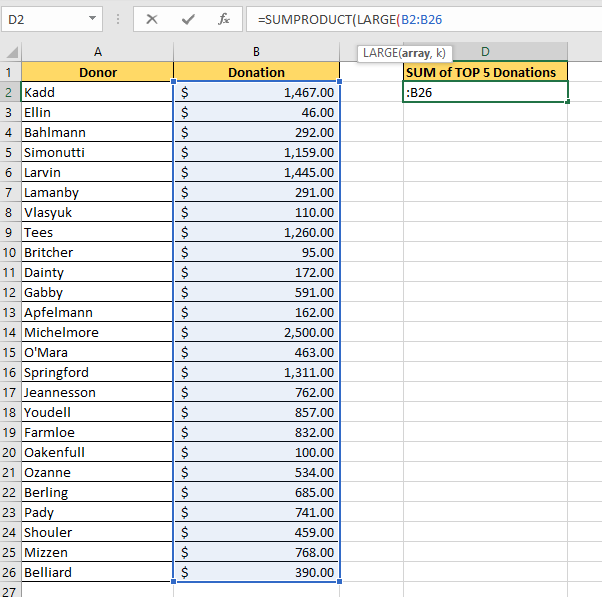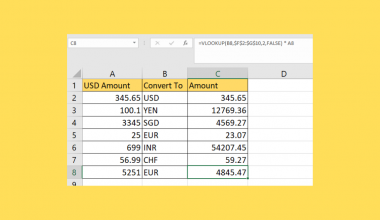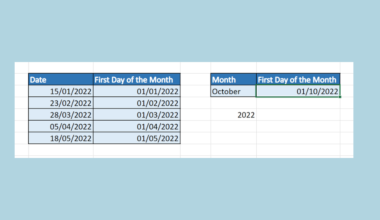# How to Find the Sum of Largest N Numbers in Excel

This guide will explain how to find the sum of the largest numbers in a given range in Excel.

We’ll use several Excel functions together to determine the summation of the largest values in a given cell range.

Let’s take a look at a simple example of where we might need to find the total sum of the top N values in a data set.

Suppose you have a list of donations for a local charity drive. You want to find out how much of the donated amount comes from the top five donors.

We can actually use the Excel functions `LARGE` and `SUMPRODUCT` to create a custom formula to achieve this.

The `LARGE` Excel function will help us find the largest n numbers in a given data set. The `SUMPRODUCT` Excel function will allow us to sum up the output of our `LARGE` function.

Now that we know when to use the `LARGE` and `SUMPRODUCT` Excel functions, let’s see how this works on an actual sample spreadsheet.

## A Real Example of Finding the Sum of the Largest N Numbers in Excel

The following section provides several examples of sheets that find the sum of the largest N numbers in Excel. We will also go into detail about the formulas and tools used in these examples.

First, let’s take a look at a spreadsheet with some sample data.

The table below shows a list of donors for a charity drive. The table also indicates how much each donor contributed to the cause.We want to know how much money was donated by the five biggest donors.To get the value in D2, we just need to use the following formula:

`=SUMPRODUCT(LARGE(B2:B26;SEQUENCE(5)))`

Let’s try to understand how the formula above works. First, we’ll use the `SUMPRODUCT` function to add a range of values. We’ve chosen the `SUMPRODUCT` function since it accepts an array of values as input.

To get our top 5 values, we use the `LARGE` and `SEQUENCE` functions together. The `LARGE` function allows you to get the Nth largest value in a certain range. We must provide a cell range with numerical values and an integer N.

Instead of a single integer N, we can provide an array of integers to get multiple results. This feature will enable us to get the 1st largest value, 2nd largest value, and so on. The `SEQUENCE` function allows us to create an array of incrementing integers of length N.

In the table below, we’ve created another table to illustrate the value of our summation given different values for N.To get the values in Column E, we’ll use the following formula:

`=SUMPRODUCT(LARGE(\$B\$2:\$B\$26;SEQUENCE(D5)))`

Do you want to take a closer look at our examples? You can make your own copy of the spreadsheet above using the link attached below.

Use our sample spreadsheet to take a closer look at the formulas we used to get the sum of the largest N values in a range.

If you’re ready to try finding the sum of the largest N numbers, head over to the next section to read our step-by-step breakdown on how to do it!

## How to Find the Sum of Largest N Numbers in Excel

This section will guide you through each step needed to find the sum of the largest N numbers in Excel. You’ll learn how to use the `SUMPRODUCT`, `LARGE`, and `SEQUENCE` functions together to sum up the largest numbers in a range.

For this guide, we will continue using the donor dataset shown in the prior section.

Follow these steps to start finding the sum of the largest N numbers in Excel:

1. First, select the cell that will hold the final result. In this example, we’ll use cell D2.2. Type ‘=SUMPRODUCT(‘ to start the `SUMPRODUCT` function.3. Type ‘LARGE(‘ to start the `LARGE` function. For the first argument, select the range that contains the list of values you want to get the top N numbers from. In this example, we’ll use the range B2:B26.4. In this example, we want to return the sum of the top five donors. If we want the `LARGE` function to return these five numbers, we must provide an array such as {1,2,3,4,5}. We can either type the array manually or with the `SEQUENCE` function.5. Hit the Enter key to evaluate the function. In our example, we find out that the top five donors contributed a total of \$7983.00 to charity.6. In the example below, we can see that we achieve the same result if we use the `SEQUENCE` function instead of using a hard-coded array.These are all the steps you need to find the sum of the largest N numbers in a given cell range.

This step-by-step guide is a quick introduction to using Excel functions to find the sum of the largest N numbers in a range.

Finding the sum of the largest N numbers is just one example of the many Excel tasks you can perform in your spreadsheets. Our website offers hundreds of other functions and methods to help you get more out of Microsoft Excel.

With so many other Excel functions available, you can find one appropriate for your use case.

Don’t miss out on our team’s new spreadsheet tips, tricks, and best practices. Subscribe to our newsletter to stay updated on the latest guides from us!### Get emails from us about Excel.

Our goal this year is to create lots of rich, bite-sized tutorials for Excel users like you. If you liked this one, you'd love what we are working on! Readers receive ✨ early access ✨ to new content.

##### You May Also Like## How to Do Currency Conversion using VLOOKUP in Excel

This guide will explain how we can perform currency conversion using the VLOOKUP function in Excel. The VLOOKUP…## How to Group Data by Week in Excel

This guide will explain how to group data by week in Excel using two simple and efficient methods. …## How to Create Multiple Folders at Once in Excel

This guide will explain how to create multiple folders at once using Microsoft Excel. When working on a…## How to Select Data for Graphs in Excel

alsoaaaThis guide will explain several ways to select data for graphs in Excel. Excel graphs are an essential…